# What’s New for Low-Code AI in MATLAB R2023a

MATLAB provides low-code apps for designing, tuning, assessing, and optimizing AI models. In this blog post, I am going to present some of the app features that were introduced in MATLAB R2023a. These new features for the Deep Network Designer, Classification Learner, Regression Learner, and Experiment apps enable more customization and integration for low-code AI.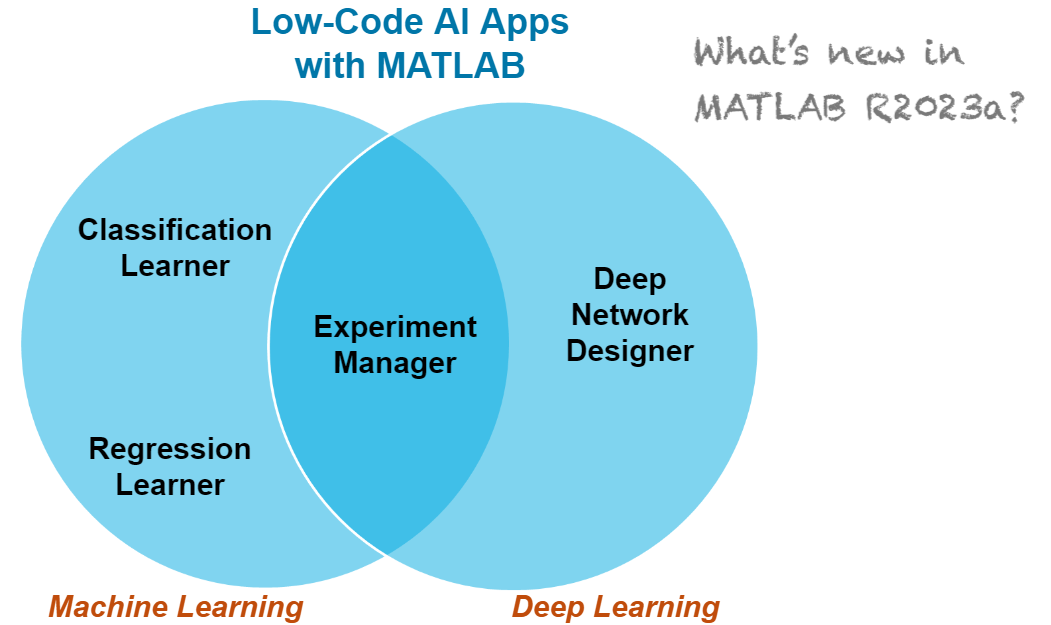Figure: MATLAB apps for low-code machine learning and deep learning   More specifically, this blog post talks about the following new features:

Deep Network Designer: View custom layers

For most deep learning tasks, you can use built-in MATLAB layers (see List of Deep Learning Layers). If there is not a built-in layer that you need for your task, then you can define you own custom deep learning layer. Another case where a network can include custom layers is when the network is imported from an external deep learning platform, such as TensorFlow™, PyTorch®, or ONNX™. The import function might generate a custom layer in place of a layer that cannot be converted to a built-in MATLAB layer. To learn more about this scenario, see our previous blog post Importing Models from TensorFlow, PyTorch, and ONNX.
######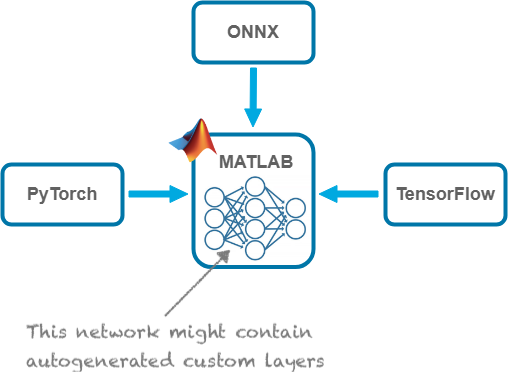Figure: Imported networks from TensorFlow, PyTorch, or ONNX might contain autogenerated custom layers.   And now you can view custom layers, autogenerated or created programmatically, in Deep Network Designer! As shown in the following figure, you can view the custom layer properties and even click on “Edit Layer Code” to open the file that contains the custom layer code.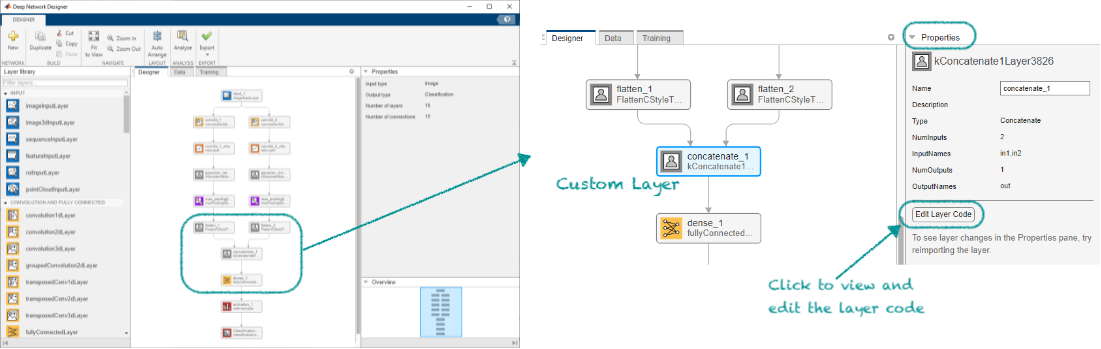Figure: View custom layer in the Deep Network Designer app.   The documentation example View Autogenerated Custom Layers Using Deep Network Designer shows how to import a model from TensorFlow and view the custom layer that is generated by the importTensorFlowNetwork function in the Deep Network Designer app. The following animation is a demonstration of this example.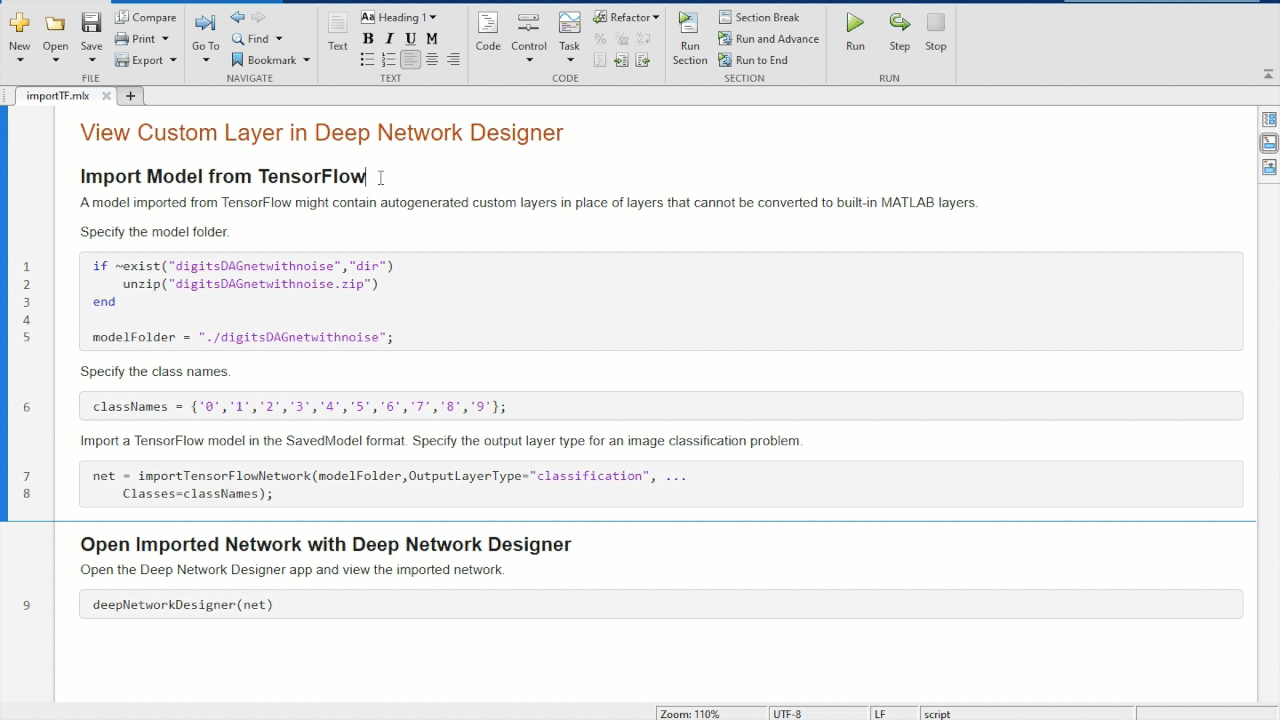Animated Figure: Import network from TensorFlow and open the network in the Deep Network Designer app to view the network architecture and autogenerated custom layer.

Deep Network Designer: Use function layers

If there is not a built-in layer that you need for your task (in addition to creating a custom layer), you can use a function layer, which applies a specified function to the layer input. Now you can use a function layer when you design networks with Deep Network Designer. You can edit the function in the app, as shown in the figure below, by directly specifying the function in the layer properties.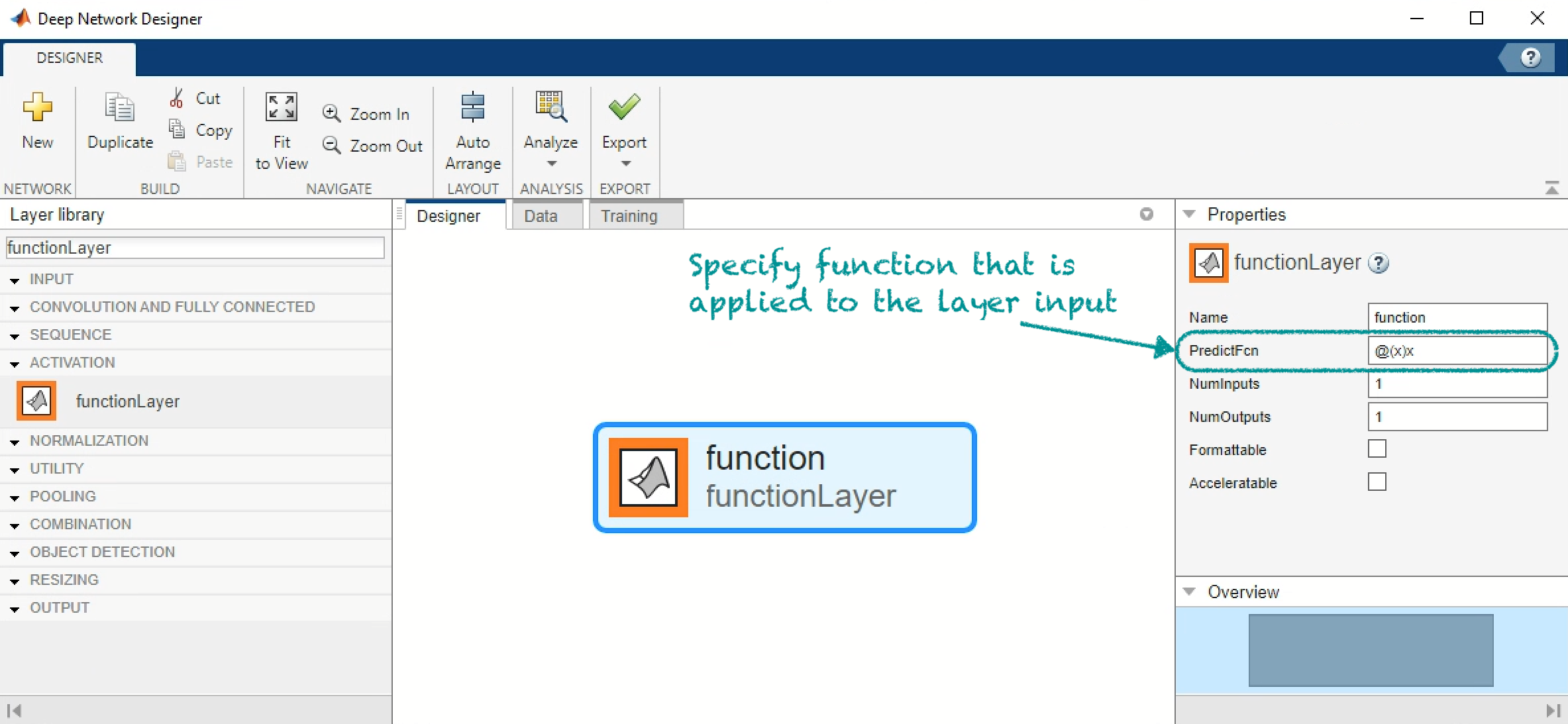Figure: Use and edit a function layer when you create a network with the Deep Network Designer app.   The following animation shows how you can create a convolutional network, which includes a function layer, with the Deep Network Designer app. The function layer applies the softsign operation ( $f(x)=\frac{x}{1+|x|}$) to the input. See the documentation example Define Softsign Layer as Function Layer to learn how to build the same network programmatically.
######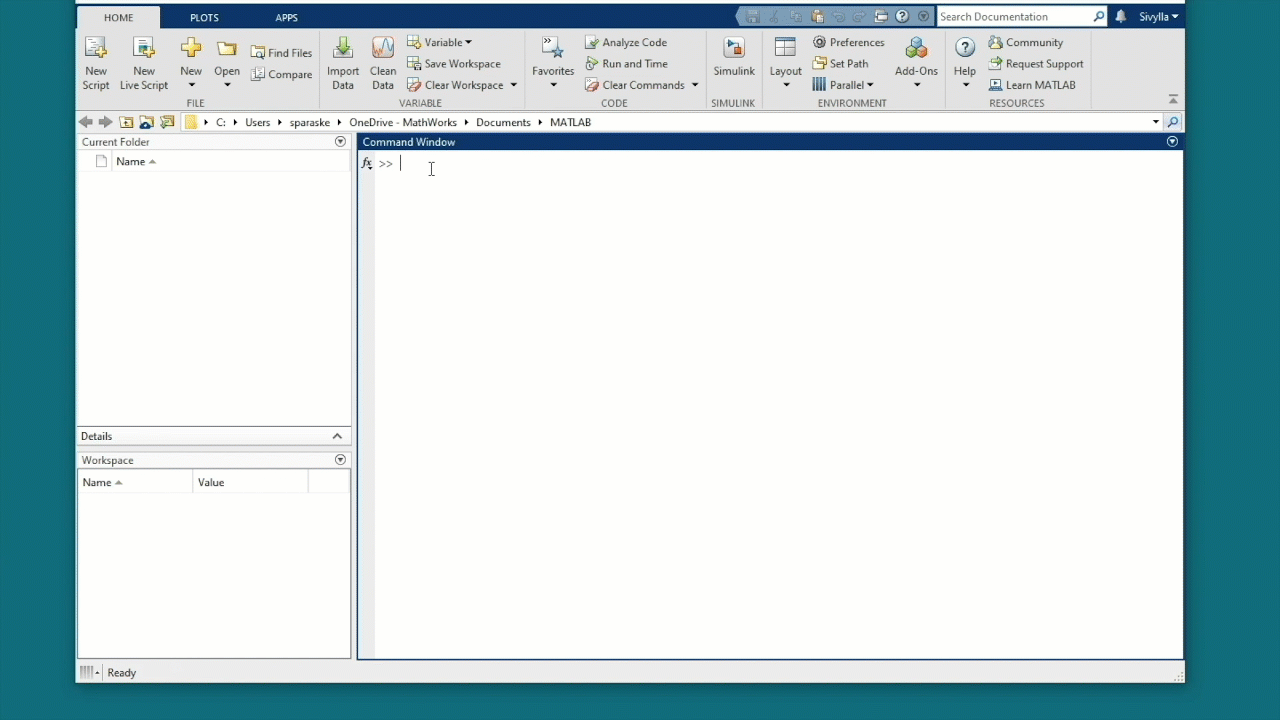Animated Figure: Use a function layer that applies the softsign operation when you build a convolution neural network in the Deep Network Designer app.

Classification Learner and Regression Learner: Export machine learning model to Experiment Manager

The Experiment Manager app was introduced 3 years ago and now you can run experiments on machine learning models in addition to deep learning models . MATLAB R2023a brings integration between machine learning apps (Classification Learner and Regression Learner) and Experiment Manager. You can now export machine learning models directly to Experiment Manager and optimize their hyperparameters. To see all tuning options, check out the documentation topics: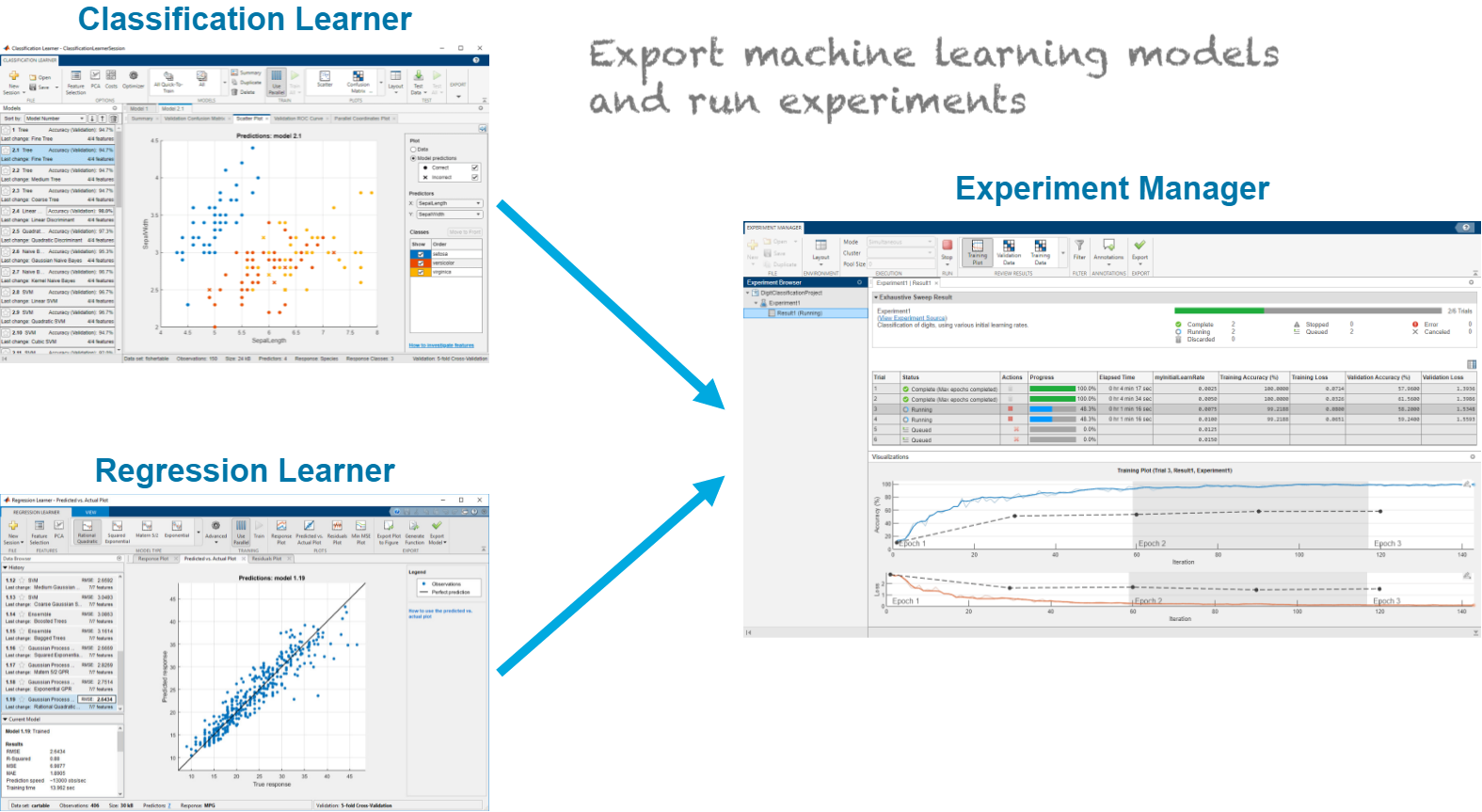Figure: Export machine learning models from the Classification Learner and Regression Learner apps to the Experiment Manager app.   You can export a trained machine learning model from the Classification Learner and Regression Learner apps by clicking on Create Experiment in the Export tab of the apps, as shown in the following figure.
######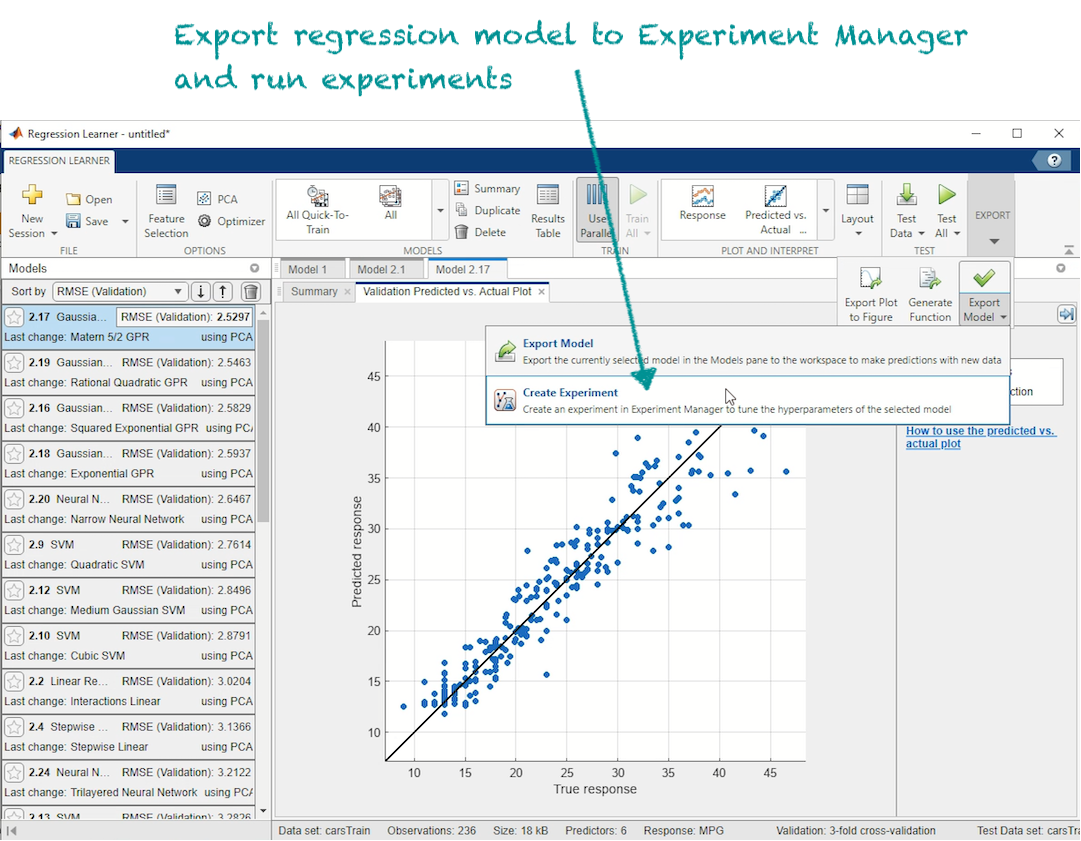Figure: Export trained regression model from Regression Learner to Experiment Manager.   The exported model is automatically loaded in a new experiment in the Experiment Manager app. Then you can run experiments on pre-selected hyperparameters, which are unique to each type of machine learning model. You can also specify which hyperparameters to run the experiment on.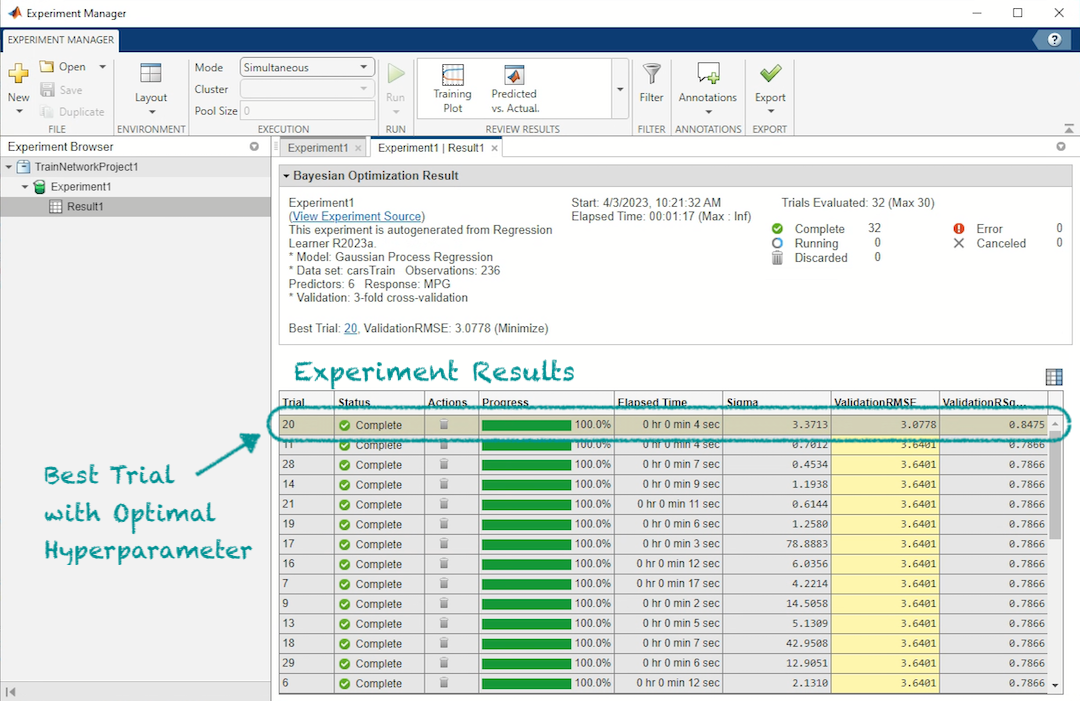Figure: Result of experiments in Experiment Manager for a model exported from Regression Learner   To see the complete workflow of how to export machine learning models and run experiments, check out the documentation examples: The following three animations show you how to perform the key steps in the example Tune Classification Model Using Experiment Manager. These steps are:
1. With a few lines of MATLAB code, prepare the data for classification.
2. In the Classification Learner app, train classification models and export the best performing model to the Experiment Manager app.
3. In the Experiment Manager app, run experiments to optimize the exported classification model’s hyperparameters.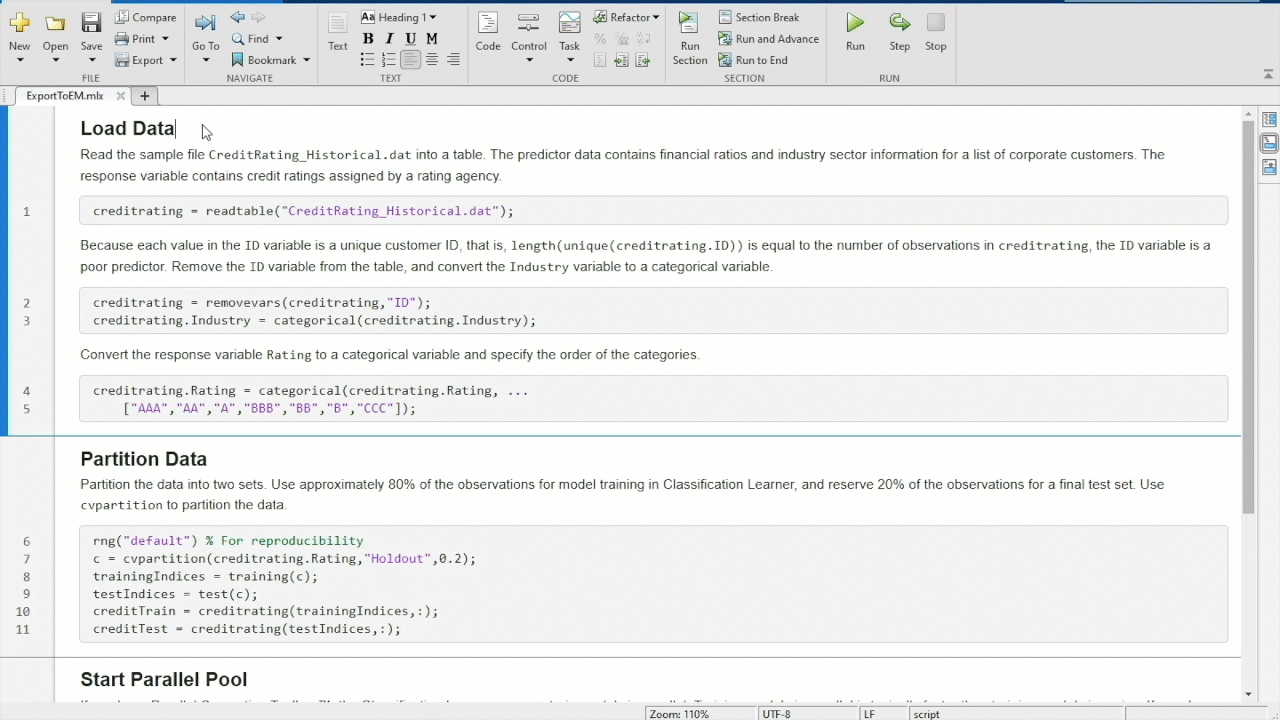Animated Figure: Prepare data for classification model and open the Classification Learner app.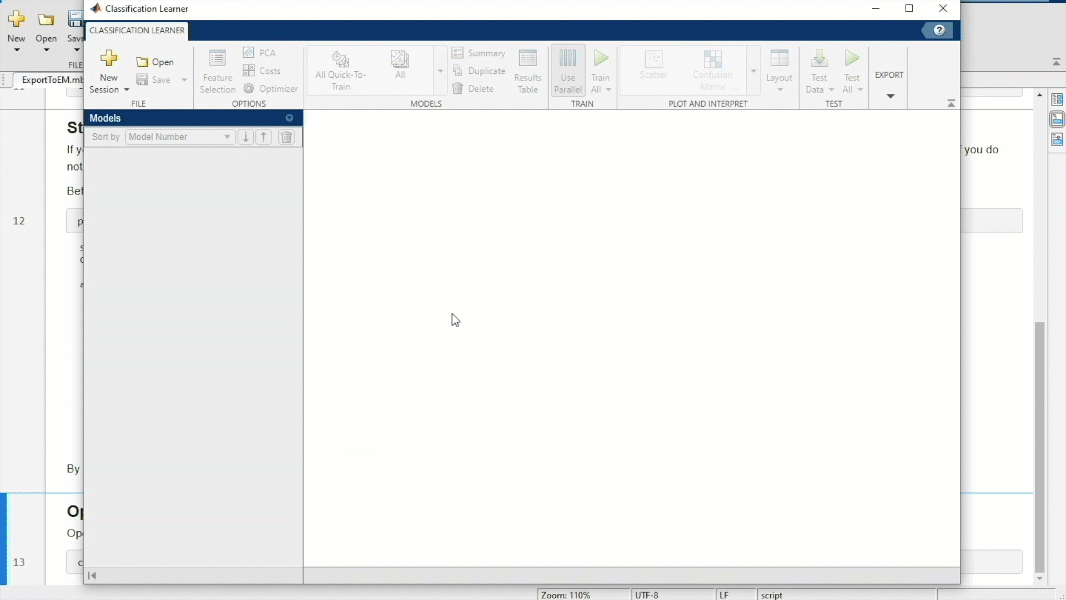Animated Figure: In the Classification Learner app, train multiple classification models, select best performing model, and export the model to the Experiment Manager app.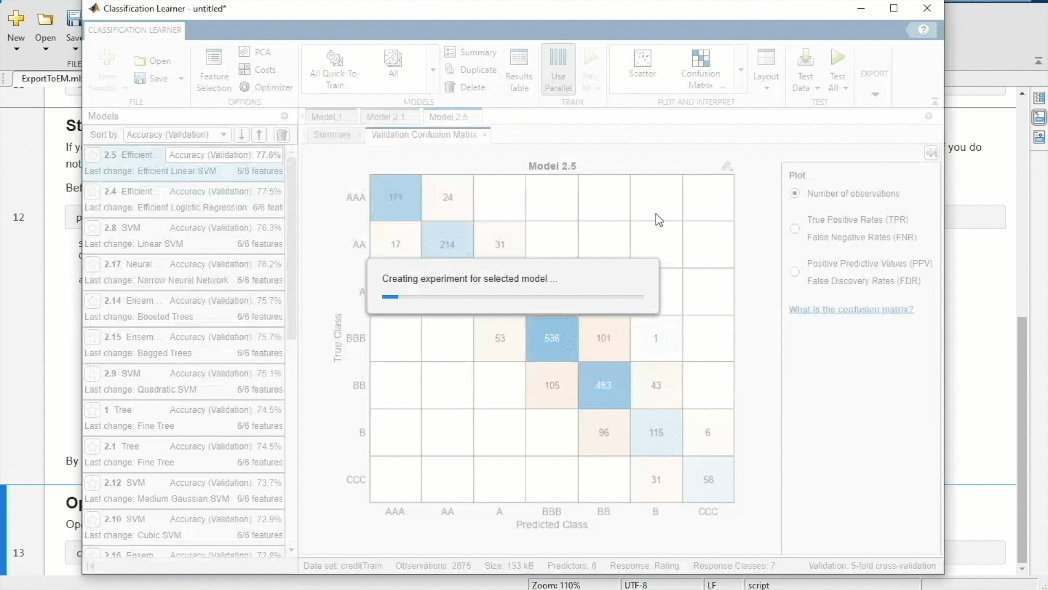Animated Figure: In the Experiment Manager app, run experiments to optimize the hyperparameters of the classification model.

Conclusion

MATLAB low-code AI apps help you get started quickly with using machine learning and deep learning, but also provide advanced features for visualization, customization, and optimization. Have you tried out one of the MATLAB AI apps? Comment here to discuss your favorite and not-so favorite app features, and how you would use the new R2023a app features. To see all the new app features, check out the machine learning and deep learning release notes.
|